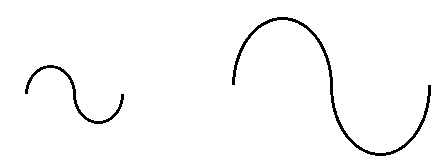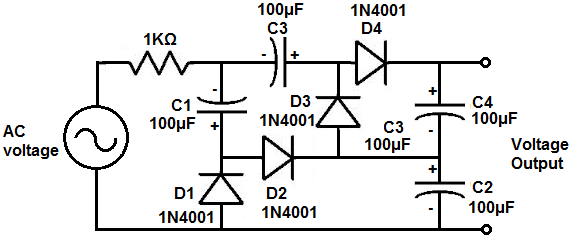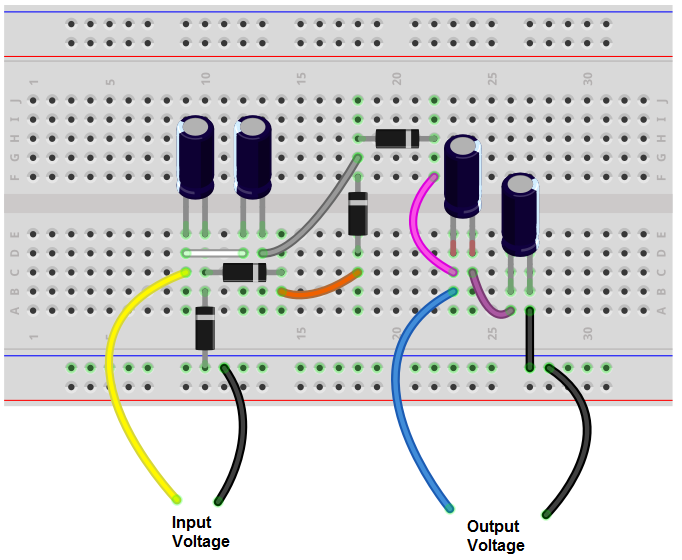﻿ How to Build a Voltage Quadrupler Circuit ﻿# How to Build a Voltage Quadrupler CircuitIn this project, we will show how to build a voltage quadrupler circuit.

A voltage quadrupler circuit is a circuit in which the output voltage is quadruple, or 4 times, the amplitude of the input voltage.

This voltage quadrupling effect is achieved through the use of capacitors. We use individuals capacitors to charge up to the input voltage. The first capacitor charges up to the input voltage of the circuit. The second capacitor has a successive effect. It charges up to the value of the input voltage but always sees the voltage from the first capacitor, having an additive effect. The result is double the input voltage. The third capacitor, again, charges up to the input voltage but sees the voltage from the other 2 capacitors, so the output is triple the input voltage. The fourth capacitor charges up to the input voltage, while seeing the voltage from the other 3 capacitors, so the output is now quadruple the input voltage. We use diodes to block capacitors from discharging once they are charged. So it's a series of capacitors and diodes that allows this multiplying effect.

The circuit intakes an input voltage and multiplies it by 4 to give an output voltage that is 4 times larger than the input voltage. Thus, this circuit is a type of voltage multiplier circuit.

The circuit intakes an AC voltage signal and outputs a larger DC voltage signal. Thus, the circuit is a type of AC-to-DC converter, an AC-to-DC boost converter.

We'll show exactly below how to connect this circuit and explain in detail how it works.

Components Needed

• 4 1N4001 Diodes
• 4 100μF electrolytic capacitors

So all that is required for this circuit are diodes and capacitors.

Really any diode can be used. It's probably better to use a diode that has a lower voltage drop across the diode than a higher one if you can. In order for a diode to work, it needs sufficient voltage dropped across it. Silicon diodes typically use about 0.7V. Other diodes, such as germanium use less, about 0.3V in order to operate. Therefore, using a lower voltage drop, the output voltage is more accurately triple the input voltage. However, it is significant especially if you are using a large input voltage, so we use silicon diodes.

The diodes are used simply for the purpose of blocking the capacitors from discharging once they charged. This is so that the diodes hodl their charge, so that the voltage at the output can be triple the voltage at the input. Since the diodes are one-way devices, current can only flow in one direction and are blocked from flowing in the other direction. Using diodes, we can allow capacitors to charge up but not discharge.

The capacitors we use all all 100μF electrolytic capacitors. Lower values can be used, such as 20-50μF, but these are hard to find, so we simply use 100μF, but you can experiment with this.

The other specification you must take into account with capacitors is the voltage rating. Depending on the input voltage you are using, you must choose the voltage ratings of the capacitors in accordance.

Since the first capacitor, C1, charges up to input voltage, its voltage rating must be a little greater than the peak of the input voltage. Since the second capacitor, C2, sees twice the voltage input voltage, its voltage rating must be twice the peak of the input voltage. Since the third capacitor, C3, sees 3 times the input voltage, its voltage rating must be 3 times the peak of the input voltage. Since the fourth capacitor, C4, sees 4 times the input voltage, its voltage rating must be 4 times the peak of the input voltage.

The voltage quadrupler circuit that we will build with capacitors and diodes is shown below.The breadboard circuit of the circuit above is shown below.So this circuit receives power from an AC voltage source such as a transformer.

Do know that this circuit works off of the basis of AC voltage.

It will not work with DC voltage.

So just realize that it must be an AC voltage source.

So at the start of the circuit, we have an AC voltage source powering the circuit.

So if we trace the path of current through this circuit, current flows from diode D1, charging up capacitor C1. Current then travels through diode D2, charging up capacitor C2. Current then goes through diode D3, charging up capacitor C3. Current then travels through diode D4, charging up capacitor C4.

So you can see that each capacitor charges up to the input voltage. Since there are 4 capacitors, this means the output voltage is 4 times the input voltage.

The first capacitor charges up to the input voltage. The second capacitor charges up to the input voltage but from the point of the anode of the capacitor, the voltages from the capacitors add. So at the point of the second capacitor, it sees the voltage from the first capacitor as well as the second one, so at this point, it's double the input voltage. Then, we have a third capacitor that charges up to the input voltage. This capacitor sees the first and second capacitor, both charged up to the input voltage, giving an output voltage that is 3 times the input voltage. We then have a fourth capacitor that charges up to the input voltage. The output voltage sees all these voltages, so the output voltage is 4 times the input voltage.

So the output voltage is directly determined by the number of capacitors. So we want quadruple the input voltage, so we use 4capacitors. In another circuit that we'll do, in which the output voltage is quadruple, or 4 times the input voltage, we use 4 capacitors.

The diodes in the circuit are used just so that we can determine how we want current to be routed in the circuit and to block the discharge of capacitors when they are charged. Since diodes are one-way electronic devices, they allow current only in one direction and prohibits current from flowing across it when reverse biased.

And this is how a voltage quadrupler circuit can be built with capacitors and diodes.

Related Resources

Voltage Doubler Circuit

Voltage Tripler Circuit